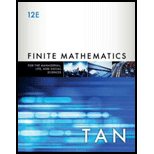Chapter 1.BMO, Problem 5BMO### Finite Mathematics for the Manager...

12th Edition
Soo T. Tan
ISBN: 9781337405782

#### Solutions

Chapter
Section### Finite Mathematics for the Manager...

12th Edition
Soo T. Tan
ISBN: 9781337405782
Textbook Problem

# Find the point of intersection of the lines 2 x − 3 y = − 2 and 9 x + 12 y = 25 .

To determine

To find:

The point of intersection of two given lines.

Explanation

Given:

The given equation of line 1 is,

2x3y=2

And,

The equation of line 2 is,

9x+12y=25

Calculation:

Consider the given equations.

2x3y=2(1)

9x+12y=25(2)

Solve the equation (1) to find the value of x.

2x3y=22x=2+3yx=3y22

Substitute 3y22 for x in equation (2).

9(3y22)+12y=2513

### Still sussing out bartleby?

Check out a sample textbook solution.

See a sample solution

#### The Solution to Your Study Problems

Bartleby provides explanations to thousands of textbook problems written by our experts, many with advanced degrees!

Get Started

## Additional Math Solutions

#### Use sets toshow that 06=0.

Mathematical Excursions (MindTap Course List)

#### Evaluate the limit, if it exists. limh01(xh)21x2h

Single Variable Calculus: Early Transcendentals, Volume I

#### Convert the expressions in Exercises 6584 to power form. 35x2

Finite Mathematics and Applied Calculus (MindTap Course List)

#### Evaluate the integral. 8. t2sintdt

Single Variable Calculus: Early Transcendentals

#### Solve for if 0360. 3sincos=1

Trigonometry (MindTap Course List)